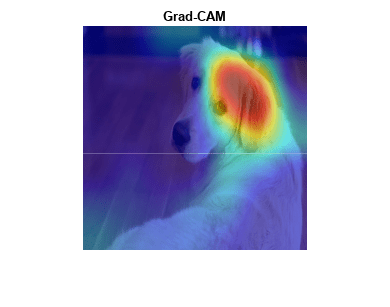# Grad-CAM Reveals the Why Behind Deep Learning Decisions

This example shows how to use the gradient-weighted class activation mapping (Grad-CAM) technique to understand why a deep learning network makes its classification decisions. Grad-CAM, invented by Selvaraju and coauthors , uses the gradient of the classification score with respect to the convolutional features determined by the network in order to understand which parts of the image are most important for classification. This example uses the GoogLeNet pretrained network for images.

Grad-CAM is a generalization of the class activation mapping (CAM) technique. This example shows Grad-CAM using the `dlgradient` automatic differentiation function to perform the required computations easily. For activation mapping techniques on live webcam data, see Investigate Network Predictions Using Class Activation Mapping.

`net = googlenet;`

### Classify Image

`inputSize = net.Layers(1).InputSize(1:2);`

Load `sherlock.jpg`., an image of a golden retriever included with this example.

`img = imread("sherlock.jpg");`

Resize the image to the network input dimensions.

`img = imresize(img,inputSize);`

Classify the image and display it, along with its classification and classification score.

```[classfn,score] = classify(net,img); imshow(img); title(sprintf("%s (%.2f)", classfn, score(classfn)));```GoogLeNet correctly classifies the image as a golden retriever. But why? What characteristics of the image cause the network to make this classification?

The idea behind Grad-CAM  is to calculate the gradient of the final classification score with respect to the final convolutional feature map. The places where this gradient is large are exactly the places where the final score depends most on the data. The `gradcam` helper function computes the Grad-CAM map for a `dlnetwork`, taking the derivative of the softmax layer score for a given class with respect to a convolutional feature map. For automatic differentiation, the input image `dlImg` must be a `dlarray`.

`type gradcam.m`
```function [featureMap,dScoresdMap] = gradcam(dlnet, dlImg, softmaxName, featureLayerName, classfn) [scores,featureMap] = predict(dlnet, dlImg, 'Outputs', {softmaxName, featureLayerName}); classScore = scores(classfn); dScoresdMap = dlgradient(classScore,featureMap); end ```

The first line of the `gradcam` function obtains the class scores and the feature map from the network. The second line finds the score for the selected classification (golden retriever, in this case). `dlgradient` calculates gradients only for scalar-valued functions. So `gradcam` calculates the gradient of the image score only for the selected classification. The third line uses automatic differentiation to calculate the gradient of the final score with respect to the weights in the feature map layer.

To use Grad-CAM, create a `dlnetwork` from the GoogLeNet network. First, create a layer graph from the network.

`lgraph = layerGraph(net);`

To access the data that GoogLeNet uses for classification, remove its final classification layer.

`lgraph = removeLayers(lgraph, lgraph.Layers(end).Name);`

Create a `dlnetwork` from the layer graph.

`dlnet = dlnetwork(lgraph);`

Specify the names of the softmax and feature map layers to use with the Grad-CAM helper function. For the feature map layer, specify either the last ReLU layer with non-singleton spatial dimensions, or the last layer that gathers the outputs of ReLU layers (such as a depth concatenation or an addition layer). If your network does not contain any ReLU layers, specify the name of the final convolutional layer that has non-singleton spatial dimensions in the output. Use the function `analyzeNetwork` to examine your network and select the correct layers. For GoogLeNet, the name of the softmax layer is `'prob'` and the depth concatenation layer is `'inception_5b-output'`.

```softmaxName = 'prob'; featureLayerName = 'inception_5b-output';```

To use automatic differentiation, convert the sherlock image to a `dlarray`.

`dlImg = dlarray(single(img),'SSC');`

Compute the Grad-CAM gradient for the image by calling `dlfeval` on the `gradcam` function.

`[featureMap, dScoresdMap] = dlfeval(@gradcam, dlnet, dlImg, softmaxName, featureLayerName, classfn);`

Resize the gradient map to the GoogLeNet image size, and scale the scores to the appropriate levels for display.

```gradcamMap = sum(featureMap .* sum(dScoresdMap, [1 2]), 3); gradcamMap = extractdata(gradcamMap); gradcamMap = rescale(gradcamMap); gradcamMap = imresize(gradcamMap, inputSize, 'Method', 'bicubic');```

Show the Grad-CAM levels on top of the image by using an `'AlphaData'` value of 0.5. The `'jet'` colormap has deep blue as the lowest value and deep red as the highest.

```imshow(img); hold on; imagesc(gradcamMap,'AlphaData',0.5); colormap jet hold off; title("Grad-CAM");```Clearly, the upper face and ear of the dog have the greatest impact on the classification.

For a different approach to investigating the reasons for deep network classifications, see `occlusionSensitivity`.

### References

 Selvaraju, R. R., M. Cogswell, A. Das, R. Vedantam, D. Parikh, and D. Batra. "Grad-CAM: Visual Explanations from Deep Networks via Gradient-Based Localization." In IEEE International Conference on Computer Vision (ICCV), 2017, pp. 618–626. Available at `Grad-CAM` on the Computer Vision Foundation Open Access website.